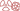THE PHENOMENON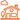IN DAILY LIFE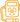QUESTION OF DOSES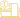AT THE DOCTOR’S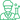At the laboratory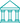At the museum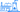NUCLEAR ENERGY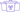A general public knowledge base dedicated to radioactivity created and maintained by the french physics community

# Stability Valley

## Beta decay : nuclei getting slimmer to achieve stability

The nucleus mass is related to its internal energy by the Einstein relation. It determines its stability. The most stable element of a series of nuclei containing the same number of nucleons is one whose mass is smaller.

The example of the nuclei family with 127 nucleons shows the relationship between weight and stability.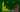At the bottom of the valley
Among the nuclei with 127 nucleons, only iodine-127 nucleus is stable. The curve representing  their nuclei mass energies (mc2), has theshape of a valley. The number of protons increases from left to right, 49 (indium) to 57 (lanthanum), while the number of neutrons decreases from 78 to 70. The energy of iodine-127 being taken as 0, the energy differences are expressed in million of electron volts. Nuclei on the left flank of the valley, in excess of neutrons regain stability through a series of beta-minus decays, those from the right side (in excess protons) by a series of beta-plus decays.

The stable element is the nucleus of iodine-127, with 53 protons and 74 neutrons. This distribution corresponds to the smallest mass nuclei in the family.

The curve of mass based on the number of protons has a parabolic shape. On the left flank, let us take into consideration the isotope of tin containing 3 more neutrons than stable iodine-127 and 3 protons less, that means 50 protons and 77 neutrons.

Because of these extra neutrons, this radioisotope has a surplus of internal energy compared to iodine-127.

Nature uses a force called the weak force to transform neutrons into protons. This process is accompanied by the production of an electron and an antineutrino. The energy needed to create an electron and an antineutrino is taken from the difference of mass energies of the father and son nuclei. This difference must be greater than the sum of the masses of the electron energies and neutrino. The neutrino mass being zero, this sum reduces to the electron mass energy, 0.511 MeV.

A beta decay costs only 0.511 MeV (510,000 electron volts) much less than the 7 to 8 million electron volts (MeV) required for the expulsion of a neutron, the competing process. Beta decay is a slow process owing to the weak force, but economical in energy.

The energy released by the transformation must exceed the threshold of 0.51 MeV to occur, a condition verified in the case of large excess of neutrons in Sn-127. This tin nucleus with 77 neutrons is thus transformed into antimony (76 neutrons), then into tellurium (75 neutrons) before reaching the stable isotope with 74 neutrons. Electrons emissions, initially very frequent, slow down as it approaches equilibrium.

Starting from the other side of the parabola with baryum-126 (56 protons), one can observe a cascade of changes of protons into neutrons, with the expulsion of positive electrons (positrons) and neutrinos. The scenario is general whatever the number of nucleons. If we add as a third dimension to the nuclear chart, the energy of mass divided by the number of nucleons, the resulting surface has the appearance of a deep valley called “valley of stability” at the bottom of which one find the most stable nuclei. One flank of the valley is populated by radioactive nuclei emitting electrons, the other by positron-emitting nuclei.

Beyond 209 nucleons, the most stable element becomes itself unstable. The nuclei become too heavy. This is the domain of alpha radioactivity.THE PHENOMENONIN DAILY LIFEQUESTION OF DOSESAT THE DOCTOR’SAt the laboratoryAt the museumNUCLEAR ENERGY